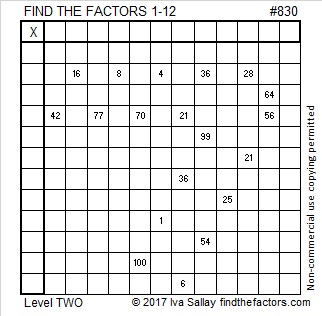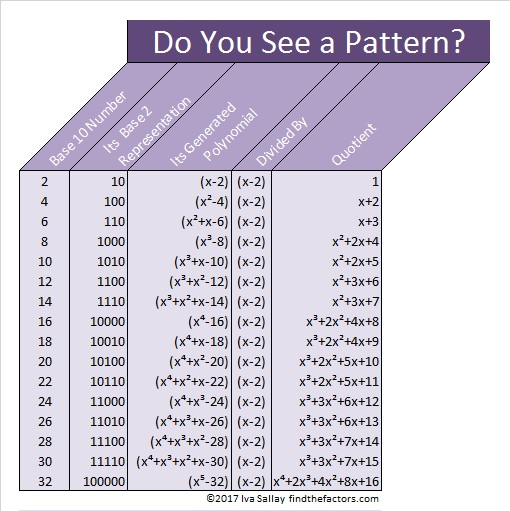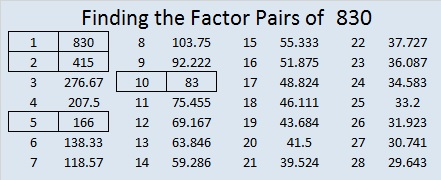# 830 I Can Divide These Polynomials By (x – 2) Without Even Looking at ThemPrint the puzzles or type the solution on this excel file: 12 factors 829-834

(x – 2) is a factor of an infinite number of polynomials. I am listing only a small, but very special subset of them here. First look for the pattern that allows us to generate a polynomial from a given number in base 2. Then look for another pattern when the polynomial is divided by (x – 2).Do you see the patterns? I do.

From the first pattern I know there is a similar special polynomial that ends with -830. AND I know from the second pattern what I will get if I divide THAT polynomial by (x – 2). Now get this: Even though I haven’t seen the polynomial yet, I know what the quotient will be! When the polynomial ending in -830 is divided by (x – 2), it will be. . . .

• x⁸ + 3x⁷ + 6x⁶ + 12x⁵ + 25x⁴ + 51x³ + 103x² + 207x + 415

And guess what, I’m right! I found the quotient without showing any steps or even looking at what I was dividing.

How did I know what that quotient would be without writing down the problem and doing some division first? Well, not only is this polynomial special, but the quotient is special, too!

All I needed to know was that the last term was -830. I then divided 830 repeatedly by 2. Any time my quotient was an odd number, I subtracted one from it before I divided it again by 2. I repeated the process until I reached zero. That is how I got all my coefficients. Even though I could do this problem without showing any work, I made a gif so you and anyone else can quickly see how I did it, but you’ll have to look sideways at it to see it. Showing steps is ALWAYS a good thing.make science GIFs like this at MakeaGif
As a side benefit, this is another way to find out what 830 is in BASE 2.

Here’s a little more about the number 830:

• 830 is a composite number.
• Prime factorization: 830 = 2 × 5 × 83
• The exponents in the prime factorization are 1, 1, and 1. Adding one to each and multiplying we get (1 + 1)(1 + 1)(1 + 1) = 2 × 2 × 2 = 8. Therefore 830 has exactly 8 factors.
• Factors of 830: 1, 2, 5, 10, 83, 166, 415, 830
• Factor pairs: 830 = 1 × 830, 2 × 415, 5 × 166, or 10 × 83
• 830 has no square factors that allow its square root to be simplified. √830 ≈ 28.80972830 is the sum of four consecutive prime numbers:

• 197 + 199 + 211 + 223 = 830

Because 5 is one of its factors, 830 is the hypotenuse of a Pythagorean triple:

• 498-664-830; that’s 166 times (3-4-5)This site uses Akismet to reduce spam. Learn how your comment data is processed.